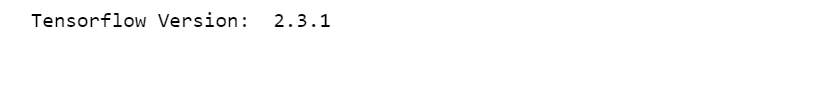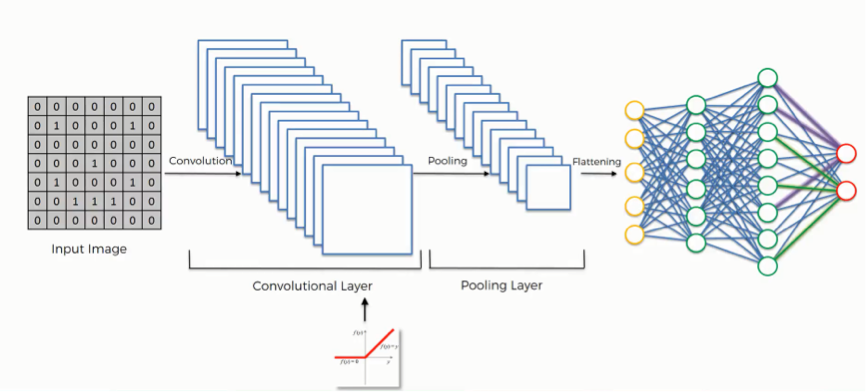1602802800

# Multiclass Classification with CNN-2D

In Multiclass classification, the instances can be classified into one of three or more classes. Here, the Dataset contains image data of Natural Scenes around the world that are distributed into 6 different categories. {‘buildings’- 0, ‘forest’- 1, ‘glacier’- 2, ‘mountain’- 3, ‘sea’ - 4, ‘street’ - 5 }

There are 14034 images in Train data and 3000 images in Test data.

### Import Libraries

``````import tensorflow
from tensorflow.keras.models import Sequential
from tensorflow.keras.layers import Conv2D
from tensorflow.keras.layers import MaxPooling2D
from tensorflow.keras.layers import Flatten
from tensorflow.keras.layers import Dense, Dropout
from tensorflow.keras.utils import to_categorical
print('Tensorflow Version: ',tensorflow.__version__)
``````### CNN-2D Model

``````## ImageDataGenerator generate batches of tensor image data with real-time data augmentation.
from tensorflow.keras.preprocessing.image import ImageDataGenerator

train_datagen = ImageDataGenerator(rescale = 1./255,
shear_range = 0.2,
zoom_range = 0.2,
horizontal_flip = True)
test_datagen = ImageDataGenerator(rescale = 1./255)
training_set= train_datagen.flow_from_directory('dataset/seg_train',
target_size = (64, 64),
batch_size = 32,
class_mode='binary')
test_set = test_datagen.flow_from_directory('dataset/seg_test',
target_size = (64, 64),
batch_size = 32,
class_mode='binary')
``````

#multiclass-classification #tensorflow #convolution-neural-net

## Buddha Community1602802800

## Multiclass Classification with CNN-2D

In Multiclass classification, the instances can be classified into one of three or more classes. Here, the Dataset contains image data of Natural Scenes around the world that are distributed into 6 different categories. {‘buildings’- 0, ‘forest’- 1, ‘glacier’- 2, ‘mountain’- 3, ‘sea’ - 4, ‘street’ - 5 }

There are 14034 images in Train data and 3000 images in Test data.

### Import Libraries

``````import tensorflow
from tensorflow.keras.models import Sequential
from tensorflow.keras.layers import Conv2D
from tensorflow.keras.layers import MaxPooling2D
from tensorflow.keras.layers import Flatten
from tensorflow.keras.layers import Dense, Dropout
from tensorflow.keras.utils import to_categorical
print('Tensorflow Version: ',tensorflow.__version__)
``````### CNN-2D Model

``````## ImageDataGenerator generate batches of tensor image data with real-time data augmentation.
from tensorflow.keras.preprocessing.image import ImageDataGenerator

train_datagen = ImageDataGenerator(rescale = 1./255,
shear_range = 0.2,
zoom_range = 0.2,
horizontal_flip = True)
test_datagen = ImageDataGenerator(rescale = 1./255)
training_set= train_datagen.flow_from_directory('dataset/seg_train',
target_size = (64, 64),
batch_size = 32,
class_mode='binary')
test_set = test_datagen.flow_from_directory('dataset/seg_test',
target_size = (64, 64),
batch_size = 32,
class_mode='binary')
``````

#multiclass-classification #tensorflow #convolution-neural-net1600939808

## Introduction

Image Classification is the technique to extract the features from the images to categorize them in the defined classes. It is a common-sense problem for the human to identify the images but, for the machine, it’s definitely not. At first, we have to train the machine with the images, and later, when we introduce the model with a new image then based on the training it will give us significant results.

In this article, we will understand how convolutional neural networks are helpful and how they can help us to improve our model’s performance. We will also go through the implementation of CNNs in PyTorch.

1. PyTorch Overview
2. What is CNN?
3. Implementation of CNNs in PyTorch.
4. Conclusion

#cnn #machine-learning #image-classification #data-science #deep-learning1593402240

## Binary and Multiclass Text Classification (auto detection in a model test pipeline)

In my previous article (Model Selection in Text Classification), I presented a way to select a model by making a comparison between classical machine learning and deep learning for the binary text classification problem.
The notebook is structured to run automatically with cross-validation all the algorithms and shows the results for the different metrics leaving the user free to select the algorithm according to his needs.

#tutorial #metrics #binary #multiclass #classification #testing1596204900

## Multiclass Classification

Where Binary Classification distinguish between two classes, Multiclass Classification or Multinomial Classification can distinguish between more than two classes.

Some algorithms such as SGD classifiers, Random Forest Classifiers, and Naive Bayes classification are capable of handling multiple classes natively. Others such as Logistic Regression or Support Vector Machine Classifiers are strictly binary classifiers. However, there are various strategies that you can use to perform multiclass classification with multiple binary classifiers.

## Techniques of Multiclass Classification

There are two Techniques of Multiclass Classification, OvO and OvR, let’s go through both these techniques one by one:

### OvR Strategy

One way to create a system that can classify the digit imsges into 10 classes (from 0 to 9) is to train 10 binary classifiers, one for each digit ( a 0 – detector, a 1 – detector, and so on). Then when you want to classify an image, you get the decision score from each classifier for that image and you select the class whose classifier outputs the highest score. This is called the one-versus-the-rest (OvR) strategy also known as one-versus-all.

### OvO Strategy

Another strategy is to train a binary classifier for every pair of digits: one to distinguish 0s and 1s, another to distinguish 0s and 2s, another for 1s and 2s, and so on. This is called the one-versus-one (OvO) strategy. If there are N classes, you need to train N × (N – 1)/2 classifiers. For the MNIST problem, this means training 45 binary classifiers. When you want to classify an image, you have to run the image through all 45 classifiers and see which class wins the most duels. The main advantage of OvO is that each classifier only needs to be trained on the part of the training set for the two classes that it must distinguish.

#by aman kharwal #data science #machine learning #multiclass classification #python1597940040

### Background

Recently I did a project wherein the target was multi-class. It was a simple prediction task and the dataset involved both categorical as well as numerical features.

For those of you who are wondering what multi-class classification is: If you want to answer in ‘0 vs 1’, ‘clicked vs not-clicked’ or ‘cat vs dog’, your classification problem is binary; if you want to answer in ‘red vs green vs blue vs yellow’ or ‘sedan vs hatch vs SUV’, then the problem is multi-class.

Therefore, I was researching suitable ways to encode the categorical features. No points for guessing, I was taken to medium articles enumerating benefits of mean target encoding and how it outperforms other methods and how you can use category_encoders library to do the task in just 2 lines of code. However, to my surprise, I found that no article demonstrated this on multi-class target. I went to the documentation of category_encoders and found that it does not say anything about supporting multi-class targets. I dug deeper, scouring through the source code and realized that the library only works for binary or continuous targets.

So I thought: “Inside of every problem lies an opportunity.” — Robert Kiposaki

Going deep, I went straight for the original paper by _Daniele Micci-Barreca _that introduced mean target encoding. Not only for regression problem, the paper gives the solution for both binary classification as well as multi-class classification. This is the same paper that category_encoders cites for target encoding as well.

While there are several articles explaining target encoding for regression and binary classification problems, my aim is to implement target encoding for multi-class variables. However, before that, we need to understand how it’s done for binary targets. In this article, I cover an overview of the paper that introduced target encoding, and show by example how target encoding works for binary problems.

#multiclass-classification #target-encoding #categorical-data #binary-classification #multi-class #data analytic